﻿

Non-existence of smooth rational curves of degree d = 13, 14, 15 con-tained in a general quintic hypersurface of P4 and in some quadric hypersurface

Abstract

Let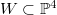$W \subset \mathbb {P}^4$ be a general quintic hypersurface. We prove that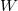$W$ contains no smooth rational curve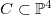$C\subset \mathbb {P}^4$ with degree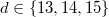$d\in \{13,14,15\}$,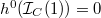$h^0(\mathcal {I} _C(1)) =0$ and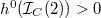$h^0(\mathcal {I} _C(2)) >0$.

DOI Code: 10.1285/i15900932v36n2p77

Keywords: General quintic $3$-fold; rational curve; Clemens' conjecture

Full Text: PDF